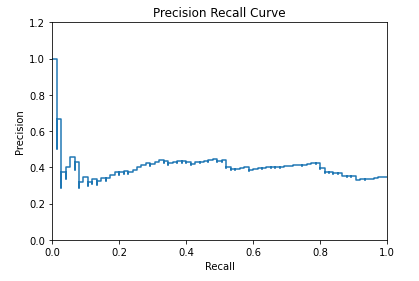....

Classification

Logistic Regression

Machine Learning

January 7, 2021

# How does the tradeoff between recall and precision work?

We know that for unbalanced datasets we can’t rely on accuracy. Here we have to measure the performance of the model using Precision & Recall. The ideal scenario would be where both Precision & Recall are high. But research has shown that the two metrics are somewhat inversely proportional to each other. I said somewhat because, Precision does decrease with increase in Recall, but beyond a certain value of Recall, Precision value becomes constant i.e. indifferent to change in recall. Following graph demonstrates the relationship beautifully:by : Monis Khan

Quick Summary:

We know that for unbalanced datasets we can’t rely on accuracy. Here we have to measure the performance of the model using Precision & Recall. The ideal scenario would be where both Precision & Recall are high. But research has shown that the two metrics are somewhat inversely proportional to each other. I said somewhat […]